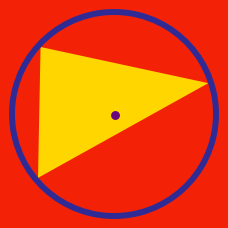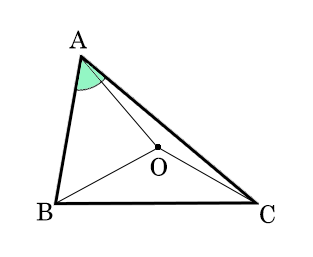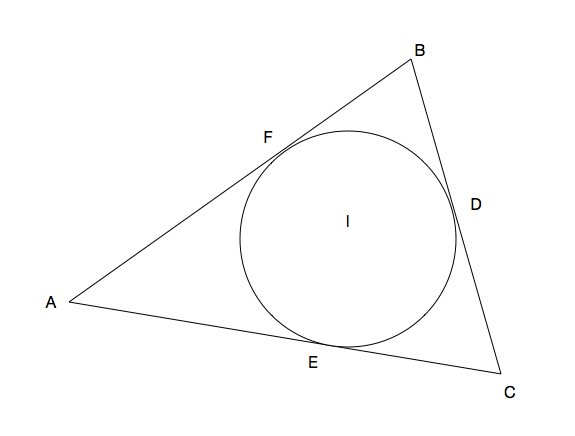Geometry

# Triangle Centers - Problem Solving

Let $A=(2,12)$, $B=(10,0)$ and $O=(0,0)$ be the vertices of triangle $AOB.$ If $G$ is the centroid of the triangle, what are the coordinates of $G$ and the area of triangle $AGB ?$Point $O$ is the circumcenter of $\triangle {ABC}$. If $\angle AOB : \angle BOC : \angle COA = 2:3:4,$ what is the measure of $\angle BAC$?Triangle $ABC$ has incenter $I$. Let the incircle be tangential to sides $AB, BC,$ and $CA$ at points $F,D,$ and $E,$ respectively. If the lengths of $BC, CA,$ and $AB$ are $19, 22,$ and $23,$ respectively, what is the length of $AF$?

$A=(17,4)$ is a vertex of triangle $ABC$ and $O=(0,0)$ is its circumcenter. $P, Q$ and $R$ are the midpoints of sides $AB, BC$ and $CA,$ respectively. If the orthocenter of triangle $PQR$ is $H,$ then what is the equation of line $AH?$

$ABC$ is an acute angle triangle with points $D$ and $E$ on $BC$ and $AC$, respectively, such that $BE$ and $AD$ are altitudes. $AD$ and $BE$ intersect at $H$. If $\angle BCA = 42 ^\circ$ and $\angle EBA = 2 \angle DAB$, what is the measure of $\angle ABC$ (in degrees)?

Details and assumptions:
- $H$ is also known as the orthocenter of the triangle, which is the intersection point of all 3 altitudes.

×